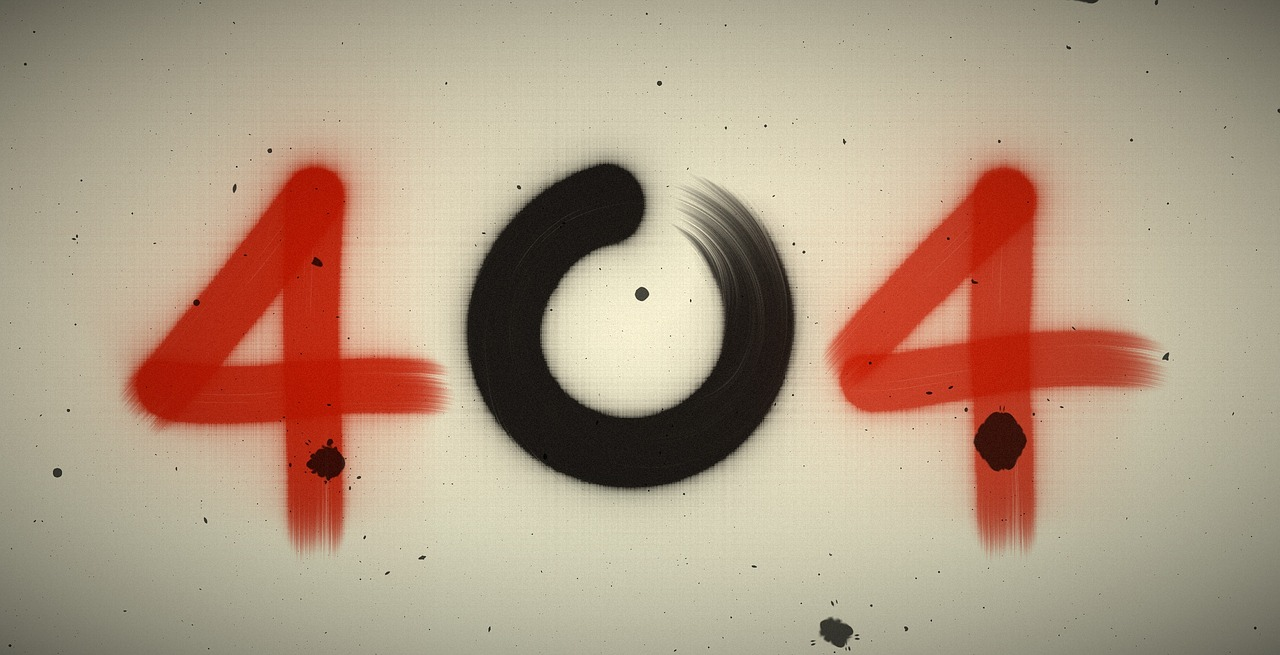## Post per Page

The 17 Equations That Changed the Course of History are The Pythagorean Theorem, Logarithms, Calculus, Law of Gravity, The square root of -1, Euler’s Polyhedra Formula, Normal distribution, Wave Equation, Fourier Transform, Navier-Stokes Equations, Maxwell’s Equations, Second Law of Thermodynamics, Relativity, Schrodinger’s Equation, Information Theory, Chaos Theory and Black-Scholes Equation.

( Image credit: Larry Phillips/Ian Stewart)

How many of these equations do you remember learning in school?

Need a refresher? Check out the descriptions of these equations here: http://bit.ly/Npi4AU

## Error Page Image# Oooops.... Could not find it!!!

The page you were looking for, could not be found. You may have typed the address incorrectly or you may have used an outdated link.